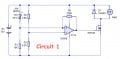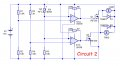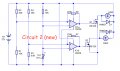# Augmenting a circuit to detect 2 levels of temperature

#### Ford Prefect

Joined Jun 14, 2010
182
Hi Guys,

I have built Circuit 1 as in the schematic below and which works extremely well.
It switches on a fan when the temperature at the thermistor (TH1) reaches about 35°C.But I wish to augment Circuit 1 and have enhanced the circuit to create Circuit 2 as below.I want the circuit to detect 2 levels of temperature which switches on FAN1 when the temperature at the thermistor (TH1) is about 40°C and then FAN2 at about 45°C.

I have at this stage only approximated the resistor values at R1,R2,R3 and R4 but I may have to do a little juggling with the values to set the temperatures I want.
But, as far as you can see, will this work and is this the correct way of doing it?
Can you think of any other (easier) way or any alterations that it might need?
Thanks

#### Dodgydave

Joined Jun 22, 2012
9,205
Last edited:
•#### AnalogKid

Joined Aug 1, 2013
8,487
As Dd said, you have interference between the two hysteresis resistors and the control voltage. When fan 1 comes on, current through R6 will shift the control voltage higher, possible enough to trip the 2nd comparator.

Reverse TH1 and R5. Now the control voltage decreases as temperature increases.
Reverse the inverting and non-i9nverting inputs of each comparator. Leave feedback resistors R6 and R7 connected to the non-inverting inputs.
Reverse R1 and R2.
Reverse R3 and R4.

The circuit action will be the same and the trip points will be the same, but now there is no common current path between the two hysteresis circuits. You will have to adjust your thinking - temperature going up makes the control voltage go down makes the 358 output go up.

ak

Last edited:
•#### Ford Prefect

Joined Jun 14, 2010
182
Yes, thank you Dodgydave and AnalogKid.
Admittedly I should have noticed this before I posted it...but it was late at night!I have reversed the components, to get this......Is there anything that I should/can do to make it better?
Eg. Do you think I should put 2 pull down resistors between the outputs of IC1/gates of Q1/Q2 and GND?

Last edited:

#### AnalogKid

Joined Aug 1, 2013
8,487
Looks good to me.

Note 1: The change in sensor voltage over 5 degrees is so small that it is possible that the two hysteresis voltage ranges might cross over if R6 and R7 are too small.

Note 2: This is complicated by the fact that the output voltage range of the 358 is not symmetrical. For a first pass approximation when calculating the two hysteresis voltage levels, you can assume the right side of R6 and R7 is either 0 V or 12 V. But in fact it's more like 0.5 V and 10.5 V. Because both of these numbers are different from the voltages across the setpoint dividers, it makes the math a bit more messy.

ak

#### Ford Prefect

Joined Jun 14, 2010
182
Looks good to me.
Note 1: The change in sensor voltage over 5 degrees is so small that it is possible that the two hysteresis voltage ranges might cross over if R6 and R7 are too small.
Note 2: This is complicated by the fact that the output voltage range of the 358 is not symmetrical. For a first pass approximation when calculating the two hysteresis voltage levels, you can assume the right side of R6 and R7 is either 0 V or 12 V. But in fact it's more like 0.5 V and 10.5 V. Because both of these numbers are different from the voltages across the setpoint dividers, it makes the math a bit more messy.

ak
Yes thanks I realise this but maybe instead of doing the math, it might be better to approximate the values and just keep testing the circuit until it works well.
But also you say 'the two hysteresis voltage ranges might cross over if R6 and R7 are too small'
don't I need to decrease the resistor values to increase the hysteresis and similarly increase the resistor values to decrease the hysteresis? Ie. If I make R6 and R7 about 50k the hysteresis will be wide whereas if I make R6 and R7 about 750k then the hysteresis will be very small?

#### AnalogKid

Joined Aug 1, 2013
8,487
Correct. It can get confusing because making the resistor small (a lower value) makes the hysteresis window large (a larger difference between the two threshold voltages). My point was just to keep an eye on things. For example (using exaggerated numbers), if the sensor voltage change over 5 degrees is 1 V, and the hysteresis window is 2 V, you will have unintended results.

ak

•Ford Prefect

#### AnalogKid

Joined Aug 1, 2013
8,487
Depends on the fan. a 7000 is rated for 200 mA continuous drain current. That's only 100 mA with margin for reliability.

ak

#### Ford Prefect

Joined Jun 14, 2010
182
Depends on the fan. a 7000 is rated for 200 mA continuous drain current. That's only 100 mA with margin for reliability.

ak
According to the specifications of the fans, they are rated at 12vDC 1.2W but at the end of the day I want to connect 4 of them (2 lots of 2 fans in parallel).

#### AnalogKid

Joined Aug 1, 2013
8,487
Assume the fan start-up current is 3x the rating for 3 seconds

ak

#### Dodgydave

Joined Jun 22, 2012
9,205
View attachment 145681

I think I may just use 2N7000 MOSFET's instead of IRF530's.
2N7000 MOSFET's should be moire than enough to handle computer fans.
Looks good, to select the correct reference resistors R1,to 4, you need to look at the datasheet for your thermistor at the temperatures you want, it will give you the resistance for those temperatures , that will give you the voltage swing with R5.